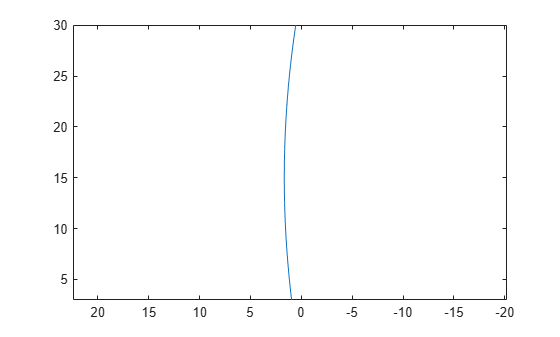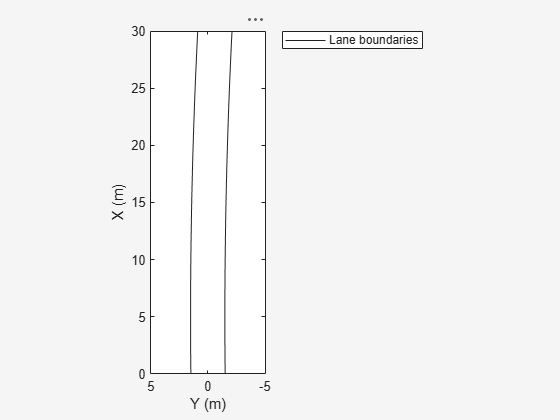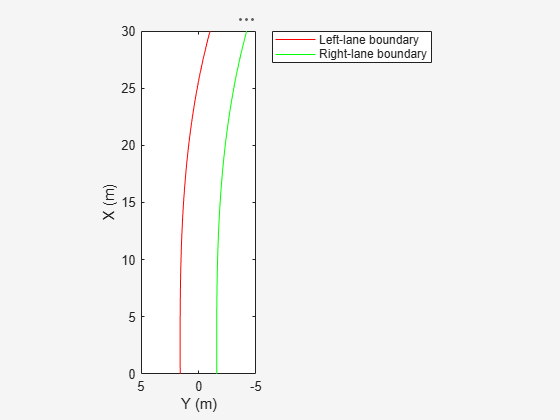# computeBoundaryModel

Obtain y-coordinates of lane boundaries given x-coordinates

## Syntax

``yWorld = computeBoundaryModel(boundaries,xWorld)``

## Description

example

````yWorld = computeBoundaryModel(boundaries,xWorld)` computes the y-axis world coordinates of lane boundary models at the specified x-axis world coordinates. If `boundaries` is a single lane boundary model, then `yWorld` is a vector of coordinates corresponding to the coordinates in `xWorld`.If `boundaries` is an array of lane boundary models, then `yWorld` is a matrix. Each row or column of `yWorld` corresponds to a lane boundary model computed at the x-coordinates in row or column vector `xWorld`. ```

## Examples

collapse all

Create a `parabolicLaneBoundary` object to model a lane boundary. Compute the positions of the lane along a set of x-axis locations.

Specify the parabolic parameters and create a lane boundary model.

```parabolicParams = [-0.005 0.15 0.55]; lb = parabolicLaneBoundary(parabolicParams);```

Compute the y-axis locations for given x-axis locations within the range of a camera sensor mounted to the front of a vehicle.

```xWorld = 3:30; % in meters yWorld = computeBoundaryModel(lb,xWorld);```

Plot the lane boundary points. To fit the coordinate system, flip the axis order and change the x-direction.

```plot(yWorld,xWorld) axis equal set(gca,'XDir','reverse')```Create a 3-meter-wide lane.

```lb = parabolicLaneBoundary([-0.001,0.01,1.5]); rb = parabolicLaneBoundary([-0.001,0.01,-1.5]);```

Compute the lane boundary model manually from 0 to 30 meters along the x-axis.

```xWorld = (0:30)'; yLeft = computeBoundaryModel(lb,xWorld); yRight = computeBoundaryModel(rb,xWorld);```

Create a bird's-eye plot and lane boundary plotter. Display the lane information on the bird's-eye plot.

```bep = birdsEyePlot('XLimits',[0 30],'YLimits',[-5 5]); lanePlotter = laneBoundaryPlotter(bep,'DisplayName','Lane boundaries'); plotLaneBoundary(lanePlotter,{[xWorld,yLeft],[xWorld,yRight]});```Create a path plotter. Create and display the path of an ego vehicle that travels through the center of the lane.

```yCenter = (yLeft + yRight)/2; egoPathPlotter = pathPlotter(bep,'DisplayName','Ego vehicle path'); plotPath(egoPathPlotter,{[xWorld,yCenter]});```Find candidate ego lane boundaries from an array of lane boundaries.

Create an array of cubic lane boundaries.

```lbs = [cubicLaneBoundary([-0.0001, 0.0, 0.003, 1.6]), ... cubicLaneBoundary([-0.0001, 0.0, 0.003, 4.6]), ... cubicLaneBoundary([-0.0001, 0.0, 0.003, -1.6]), ... cubicLaneBoundary([-0.0001, 0.0, 0.003, -4.6])];```

For each lane boundary, compute the y-axis location at which the x-coordinate is 0.

```xWorld = 0; % meters yWorld = computeBoundaryModel(lbs,0);```

Use the computed locations to find the ego lane boundaries that best meet the criteria.

```leftEgoBoundaryIndex = find(yWorld == min(yWorld(yWorld>0))); rightEgoBoundaryIndex = find(yWorld == max(yWorld(yWorld<=0))); leftEgoBoundary = lbs(leftEgoBoundaryIndex); rightEgoBoundary = lbs(rightEgoBoundaryIndex);```

Plot the boundaries using a bird's-eye plot and lane boundary plotter.

```bep = birdsEyePlot('XLimits',[0 30],'YLimits',[-5 5]); lbPlotter = laneBoundaryPlotter(bep,'DisplayName','Left-lane boundary','Color','r'); rbPlotter = laneBoundaryPlotter(bep,'DisplayName','Right-lane boundary','Color','g'); plotLaneBoundary(lbPlotter,leftEgoBoundary) plotLaneBoundary(rbPlotter,rightEgoBoundary)```## Input Arguments

collapse all

Lane boundary models containing the parameters used to compute the y-axis coordinates, specified as a lane boundary object or an array of lane boundary objects. Valid objects are `parabolicLaneBoundary` and `cubicLaneBoundary`.

x-axis locations of the boundaries in world coordinates, specified as a real scalar or real-valued vector.

## Extended Capabilities

### Functions

Introduced in R2017a